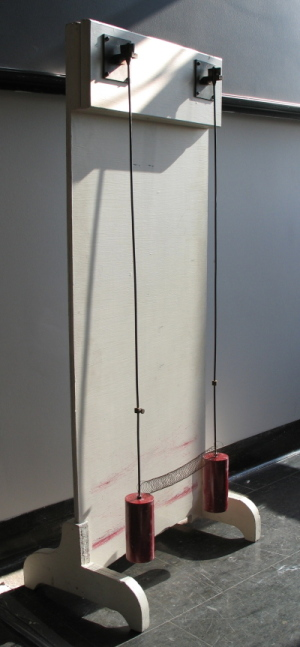M A T H 2 1 B
Mathematics Math21b Spring 2008
Linear Algebra and Differential Equations
Exhibit: double pendulum
Office: SciCtr 434## Pendulum

The pendulum motion is described by the differential equation
``` x''(t) = -sin(x)
```
where x(t) is the angle at time t. For small x, the linearization
``` x''(t) = -x
```
is a good approximation.

## Double pendulum

The picture shows the double pendulum borrowed from the Harvard lecture demonstrations. The coupled system of two pendula is
``` x''(t) = - x + c (y-x)
y''(t) = - y - c (y-x)
```
The constant c is determined by the spring strength.

## Solving the system

This system in vector form is
``` v''(t) = A v(t)
```
with the matrix
```A  = | -(1+c)   c    |
|  c     -(1+c) |
```
This matrix has the eigenvalues L1 = -1 with eigenvector v2 = [1,1]T and the eigenvalue L2 = -1-2c with the eigenvector [1,-1]T.

Write the initial position v(0) = [x(0),y(0)]T and initial velocity v'(0) = [x'(0),y'(0)]T as linear combinations of eigenvectors.
```v(0)  = a1 v1 + a2 v2
v'(0) = b1 v1 + b2 v2
```
This produces the solution
```v(t)  = [ a1 cos(t) + b1 sin(t) ] v1
+ [ a2 cos((1+2c)1/2 t) + b2 sin((1+2c)1/2 t) ] v2
```

## Eigenmodes

In this system we can "see" the eigenvalues as the squared frequencies of the eigenmodes. One can also see the eigenmodes.
1) Starting with an initial condition where bi=0 and a2=0, we have a double pendulum where both pendula swing parallel. The spring strenght does not matter and the penduli move as if they were not connected.
2) Starting with an initial condition where bi=0 and a1=0, the pendulum swing against each other. The frequency (1+2c)1/2 has become slightly larger.
 The first eigenmode. The second eigenmode. It swings a bit faster. The small spring strength makes it swing only slightly faster. A general initial condition.